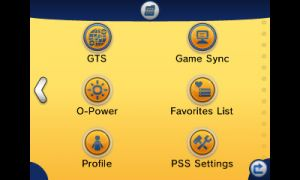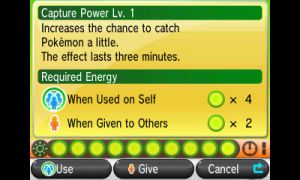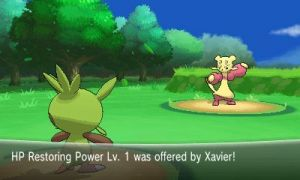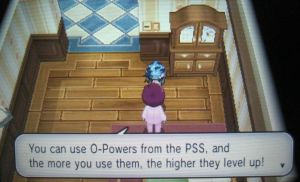# O-Powers

Pokemon X Walkthrough and Guide by Paul (vhayste)
Thank you for printing this page from www.SuperCheats.com. Remember to come back to check for updates to this guide and much more content for Pokemon X

## O-Powers

Last Updated:
O-Powers are bonuses that you can give another player or use it on yourself, similar to Pokemon Black and White. These powers give various bonuses ranging from increased stats, experience points, money or even boosting the hatching speed or encounter rate. The effects last for three minutes however, you can make them more powerful and efficient by increasing their levels up to LV3. You can increase the levels by regularly using the powers or giving them to other players. The exception are the Encounter or Stealth powers. They don't have any levels and you can't give them to other players as well.

You spend energy points to use an O-Power which can be replenished over time. The rate on how the energy point is recovered depends on the number of steps you made in a day.

O-Power Recovery Rate Multiplier
x1: 0-1999 steps
x2: 2000-2999
x3: 3000-3999
x4: 4000 aboveYou can get new O-Powers by talking to Mr. Bonding. He usually hangs out in different hotels or Pokemon Centers.HP Restoring Power Recovers some HP to a Pokemon in battle Effect LV1 = +20, LV2 = +50, LV3 = +200 Friend Energy Cost LV1 = 1, LV2 = 2, LV3 = 3 Self Energy Cost LV1 = 2 , LV2 = 3, LV3 = 4 Location Default

 Capture Power Increases the chances of capturing wild Pokemon Effect LV1 = +10%, LV2 = +20%, LV3 = +30% Friend Energy Cost LV1 = 2, LV2 = 3, LV3 = 4 Self Energy Cost LV1 = 4 , LV2 = 5, LV3 = 6 Location Default

 Bargain Power Lowers the prices of Pokemart items Effect LV1 = -10%, LV2 = -25%, LV3 = -50% Friend Energy Cost LV1 = 1, LV2 = 2, LV3 = 3 Self Energy Cost LV1 = 2 , LV2 = 3, LV3 = 4 Location Lumiose City - Hotel Richissime

 Attack Power Raises the Pokemon's attack in battle Effect LV1 = 1 stage, LV2 = 2 stages, LV3 = 3 stages Friend Energy Cost LV1 = 1, LV2 = 2, LV3 = 3 Self Energy Cost LV1 = 3 , LV2 = 4, LV3 = 5 Location Route 5 Gate

 Defense Power Raises the Pokemon's defense in battle Effect LV1 = 1 stage, LV2 = 2 stages, LV3 = 3 stages Friend Energy Cost LV1 = 1, LV2 = 2, LV3 = 3 Self Energy Cost LV1 = 3 , LV2 = 4, LV3 = 5 Location Route 5 Gate

 Sp. Atk. Power Raises the Pokemon's special attack in battle Effect LV1 = 1 stage, LV2 = 2 stages, LV3 = 3 stages Friend Energy Cost LV1 = 1, LV2 = 2, LV3 = 3 Self Energy Cost LV1 = 3 , LV2 = 4, LV3 = 5 Location Camphier Hotel

 Sp. Def. Power Raises the Pokemon's special defense in battle Effect LV1 = 1 stage, LV2 = 2 stages, LV3 = 3 stages Friend Energy Cost LV1 = 1, LV2 = 2, LV3 = 3 Self Energy Cost LV1 = 3 , LV2 = 4, LV3 = 5 Location Ambrette Hotel

 Accuracy Power Raises the Pokemon's Accuracy in battle Effect LV1 = 1 stage, LV2 = 2 stages, LV3 = 3 stages Friend Energy Cost LV1 = 1, LV2 = 2, LV3 = 3 Self Energy Cost LV1 = 3 , LV2 = 4, LV3 = 5 Location Dendemile Town Pokemon Center

 Speed Power Raises the Pokemon's speed in battle Effect LV1 = 1 stage, LV2 = 2 stages, LV3 = 3 stages Friend Energy Cost LV1 = 1, LV2 = 2, LV3 = 3 Self Energy Cost LV1 = 3 , LV2 = 4, LV3 = 5 Location Geosenge Hotel

 Critical Power Raises the Pokemon's critical rate in battle Effect LV1 = +1, LV2 = +2, LV3 = +3 Friend Energy Cost LV1 = 1, LV2 = 2, LV3 = 3 Self Energy Cost LV1 = 2 , LV2 = 3, LV3 = 4 Location Shalour City - Pokémon Center

 Experience Point Power Increases the experience point obtained within the time limit. Effect LV1 = +20%, LV2 = +50%, LV3 = +100% Friend Energy Cost LV1 = 2, LV2 = 3, LV3 = 4 Self Energy Cost LV1 = 4, LV2 = 5, LV3 = 6 Location Anistar City Pokemon Center

 Prize Money Power Increases the prize money obtained from winning trainer battles within the time limit. Effect LV1 = +50%, LV2 = +100%, LV3 = +200% Friend Energy Cost LV1 = 2, LV2 = 3, LV3 = 4 Self Energy Cost LV1 = 4, LV2 = 5, LV3 = 6 Location Cyllage City Hotel

 Befriending Power Increases your Pokemon's happiness by a certain amount. Effect LV1 = x1, LV2 = x2, LV3 = x3 Friend Energy Cost LV1 = 1, LV2 = 2, LV3 = 3 Self Energy Cost LV1 = 2, LV2 = 3, LV3 = 4 Location Coumarine City Pokemon Center

 Encounter Power Increases the chances of encountering wild pokemon Effect LV1 = 50%, LV2 = 100%, LV3 = +200% Friend Energy Cost N/A Self Energy Cost LV1 = 2, LV2 = 3, LV3 = 4 Location Laverre City Pokémon Center

 Stealth Power Decreases the chances of encountering wild pokemon Effect LV1 = 0.6%, LV2 = 0.5%, LV3 = 0.3% Friend Energy Cost N/A Self Energy Cost LV1 = 2, LV2 = 3, LV3 = 4 Location Couriway Town Hotel

 PP Restoring Power Recovers a bit of your Pokemon's PP Effect LV1 = +5, LV2 = +10, LV3 = +40 Friend Energy Cost LV1 = 1, LV2 = 2, LV3 = 3 Self Energy Cost LV1 = 2, LV2 = 3, LV3 = 4 Location Snowbelle City

 Hatching Power Reduces the amount of time needed to hatch an egg Effect N/A Friend Energy Cost LV1 = 1, LV2 = 2, LV3 = 3 Self Energy Cost LV1 = 2, LV2 = 3, LV3 = 4 Location Lumiose City - Max Style required

Verdict:

Anything missing from this guide?
for Pokemon X